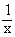Home MonkeyNotes Printable Notes Digital Library Study Guides Study Smart Parents Tips College Planning Test Prep Fun Zone Help / FAQ How to Cite New Title Request

4.10 Derivative of An Inverse Function

In algebra x-1 is also written asand it is the reciprocal of x. However in trigonometry, the -1 has a different meaning, and cos-1x stands for the angle whose cosine is x. Now -1 becomes the part of the symbol cos. Similarly sin-1x is the angle whose sine is x. However there are infinite number of angles which have same sine, cosine and tangent, etc. It is usual to consider only their principal values. Thus each of the six basic trigonometric functions have corresponding inverses when appropriate restrictions are placed on the domain of their original functions. All these inverse functions have derivatives.

THEOREM : If y = f (x) is a derivable function of x such that the inverse function x = f -1 (y) is defined thenYour browser does not support the IFRAME tag.

Index

4. 1 Derivability At A Point
4. 2 Derivability In An Interval
4. 3 Derivability And Continuity Of A Function At A Point
4. 4 Some Counter Examples
4. 5 Interpretation Of Derivatives
4. 6 Theorems On Derivatives (differentiation Rules)
4. 7 Derivatives Of Standard Functions
4. 8 Derivative Of A Composite Function
4. 9 Differentiation Of Implicit Functions

4.10 Derivative Of An Inverse Function
4.11 Derivatives Of Inverse Trigonometric Functions
4.12 Derivatives Of Exponential & Logarithmic Functions
4.13 Logarithmic Differentiation
4.14 Derivatives Of Functions In Parametric Form
4.15 Higher order Derivatives

Chapter 5